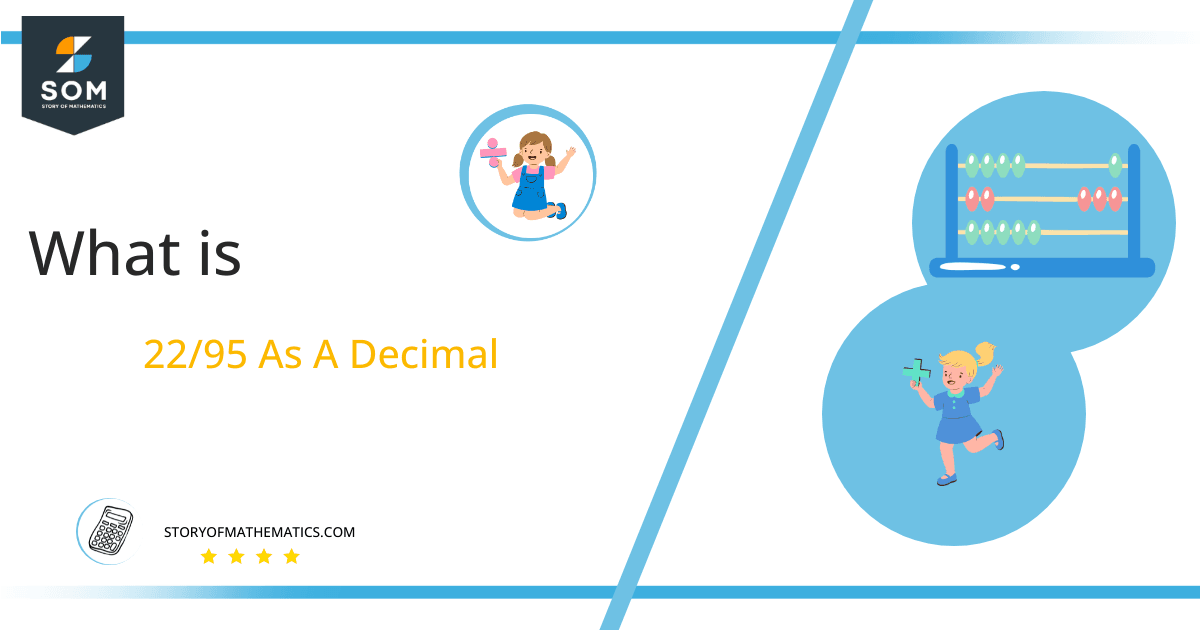# What Is 22/95 as a Decimal + Solution With Free Steps

The fraction 22/95 as a decimal is equal to 0.231.

The division of two numbers can be represented in the form of a fraction p/q. All rational numbers can also be represented in the form of a fraction. Therefore, the result of a fraction can be an integer or a decimal value, depending on p and q. If p > q, the fraction is called an improper fraction, and otherwise a proper fraction.

Here, we are more interested in the division types that result in a Decimal value, as this can be expressed as a Fraction. We see fractions as a way of showing two numbers having the operation of Division between them that result in a value that lies between two Integers.Now, we introduce the method used to solve said fraction to decimal conversion, called Long Division, which we will discuss in detail moving forward. So, let’s go through the Solution of fraction 22/95.

## Solution

First, we convert the fraction components, i.e., the numerator and the denominator, and transform them into the division constituents, i.e., the Dividend and the Divisor, respectively.

This can be done as follows:

Dividend = 22

Divisor = 95

Now, we introduce the most important quantity in our division process: the Quotient. The value represents the Solution to our division and can be expressed as having the following relationship with the Division constituents:

Quotient = Dividend $\div$ Divisor = 22 $\div$ 95

This is when we go through the Long Division solution to our problem.## 22/95 Long Division Method

We start solving a problem using the Long Division Method by first taking apart the division’s components and comparing them. As we have 22 and 95, we can see how 22 is Smaller than 95, and to solve this division, we require that 22 be Bigger than 95.

This is done by multiplying the dividend by 10 and checking whether it is bigger than the divisor or not. If so, we calculate the Multiple of the divisor closest to the dividend and subtract it from the Dividend. This produces the Remainder, which we then use as the dividend later.

Now, we begin solving for our dividend 22, which after getting multiplied by 10 becomes 220.

We take this 220 and divide it by 95; this can be done as follows:

220 $\div$ 95 $\approx$ 2

Where:

95 x 2 = 190

This will lead to the generation of a Remainder equal to 220 – 190 = 30. Now this means we have to repeat the process by Converting the 30 into 300 and solving for that:

300 $\div$ 95 $\approx$ 3

Where:

95 x 3 = 285

This, therefore, produces another Remainder which is equal to 300 – 285 = 15. Now we must solve this problem to Third Decimal Place for accuracy, so we repeat the process with dividend 150.

150 $\div$ 95 $\approx$ 1

Where:

95 x 1 = 95

Finally, we have a Quotient generated after combining the three pieces of it as 0.231, with a Remainder equal to 55.Images/mathematical drawings are created with GeoGebra.